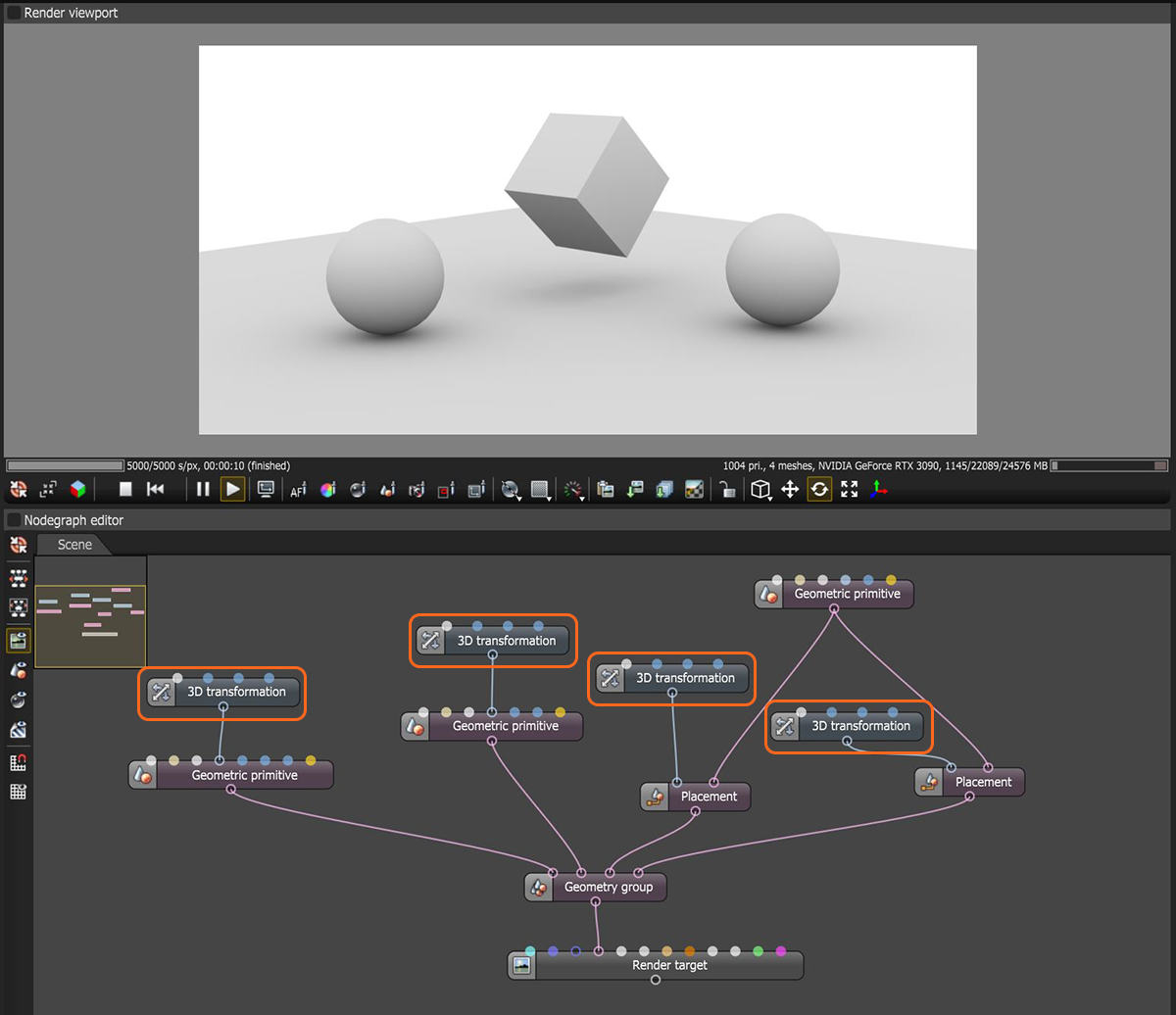# Transforms

A Transform is the numerical representation of an Object geometry instance, where the object may be any of the following:

• Mesh
• Volume
• Geometric Primitive
• Placement node
• Scatter node
• Group node

Transform nodes can be used to layout scene objects or relocate imported scene objects (figure 1).Figure 1: A Transform node connected to a series of Placement nodes and geometric primitives

When applied to a Scatter node, the number of transformed instances is almost unlimited, and are represented as a list of transforms. See the Scatter topic in this manual for information about the Scatter node.

There are five types of transform nodes:

• 2D Transform
• 3D Rotation
• 3D Scale
• 3D Transform
• Transform Value

Each of these are covered in more detail in the Transforms topic.